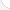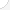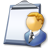Surveyors, cartographers, photogrammetrists, and surveying technicians Description: These workers measure and map the earth's surface to set official land, air, and water boundaries. They may check old legal documents for information and write reports. They work outdoors in all kinds of weather and may travel long distances to work sites. Cartographers use the information surveyors gather to prepare maps and charts.Source: Bureau of Labor Statistics, U.S. Department of Labor, Occupational Outlook Handbook, 2008-09 Edition at http://www.bls.gov/OCO/Complete Job Profile: http://www.bls.gov/oco/ocos040.htmSalary: \$28,001 to \$50,000 per yearComments:

There are 41 math topics Surveyors, cartographers, photogrammetrists, and surveying technicians need to know.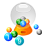Basic Math / Algebra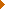DecimalsRatio and ProportionPercentCustomary MeasurementMetric MeasurementMeasurement ConversionBasic ProbabilityBasic StatisticsPowers and RootsNegative NumbersBasic Problem Solving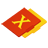First-Year AlgebraUsing FormulasLinear EquationsCoordinate Graphing 2DAlgebraic Representation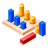GeometryBasic TerminologyAngle MeasurementCongruent TrianglesTriangle InequalitiesParallel LinesQuadrilateralsSimilarityPythagorean TheoremRight Triangle TrigonometryCirclesConstructionsAreaVolumeTransformationsMake/Use 3D Drawings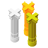Second-Year Algebra / TrigonometryAdvanced StatisticsTrigonometric/Circular FunctionsOblique TrianglesPolar Coordinates/Graphs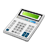Other TopicsBasic Calculator UseScientific Calculator UseComputer UseGroup Problem SolvingMental MathInductive/Deductive ReasoningMathematical Modeling